﻿Poster

# Poster

This mode enables you to enlarge an original to a size larger than A3 by copying parts of the original over several sheets of paper, and then pasting the sheets together.
You can specify the copy ratio for the Poster mode by percentage, or by setting the number of output pages that the original image should be copied over.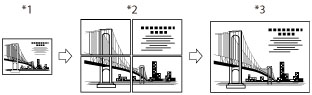*1 Original
*2 Copy
*3 Copies Cut and Pasted Together
##### Determining the Copy Ratio and Number of Sheets Needed
If you create a poster by specifying the copy ratio, the number of output pages changes according to the size of the original, the copy ratio setting, and the size of the copy paper. Refer to the following table to determine the desired copy ratio.
 Output size Original size Optimum paper size and sheet quantity A3 B4 A4 A0 x 2 (horizontal) 400% - - B4 5 x 5 = 25 sheets B0 (horizontal) 346% 400% - A3 4 x 4 = 16 sheets A0 (horizontal) 282% 326% - A3 3 x 3 = 9 sheets B1 (horizontal) 244% 282% - B4 3 x 3 = 9 sheets A1 (horizontal) 200% 230% - B4 3 x 3 = 9 sheets B2 (horizontal) 173% 200% - A3 2 x 2 = 4 sheets A2 (horizontal) 141% 163% - B4 2 x 2 = 4 sheets B3 (horizontal) 122% 141% - A4R 2 x 2 = 4 sheets A0 (vertical) - - 400% B4 3 x 5 = 15 sheets B1 (vertical) - - 346% A3 2 x 4 = 8 sheets A1 (vertical) - - 282% A3 2 x 3 = 6 sheets B2 (vertical) - - 244% B4 2 x 3 = 6 sheets A2 (vertical) - - 200% A3 1 x 3 = 3 sheets B3 (vertical) - - 173% A3 1 x 2 = 2 sheets
 NOTE The sizes indicated in the table serve only as a guideline. The actual copy ratio may be different from that indicated in the table.After all the sheets have been pasted together, the final size of the actual copy will be slightly smaller than the size indicated in the table.You can create a poster with copy ratios that are not listed in the table. However, the size of the original must not be larger than A3, and the copy ratio cannot exceed 400%.
##### Pasting the Sheets of the Divided Image Together
Example: Enlarging an A4 original by 282% to create an A1 vertical copy.
 Size of Original: A4 Orientation of Original: Vertical Copy Size: A1 Orientation of Copy: Vertical
Based on the above criteria, the table under "Determining the Copy Ratio and Number of Sheets Needed" indicates that when using A3 paper, six pages (2 x 3) are output.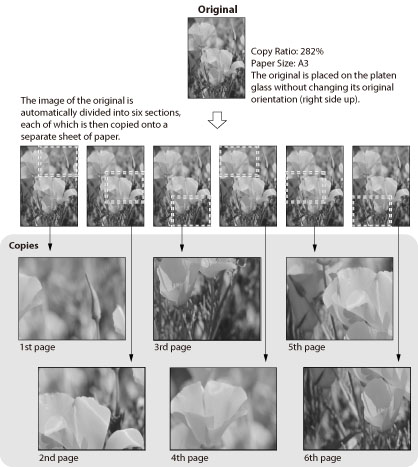The images on neighboring sheets created by the Poster mode overlap slightly. When pasting the sheets together, trim the overlapping portions, leaving an adequate amount of overlap to apply the glue.
The width of the overlapping portions differs, according to the copy ratio and paper size.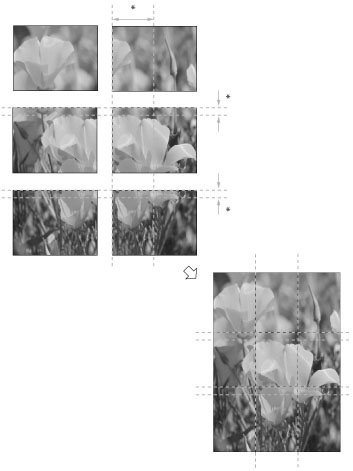*Overlap
 IMPORTANT Place your original on the platen glass. The Poster mode cannot be used if the original is placed in the feeder.If you set the Poster mode while the color mode is set to 'Auto (Color/B&W)', the color mode is automatically set to 'Full Color'.The Poster mode cannot be used to make copies on Custom Size paper, envelopes, transparencies/clear film, and tab paper.
 NOTE Copies of the original image made in the Poster mode overlap. Join neighboring sheets together by pasting over the overlapping areas.You cannot make more than one copy of the original (over multiple sheets of paper) at one time using the Poster mode.To cancel this setting, press [Copy Ratio] → [Poster] → [Cancel].

## Specifying the Copy Ratio in Percentage

You can enlarge an original in the Poster mode by specifying the copy ratio in percentage. Any copy ratio from 100% to 400% can be set.
1.
Press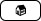→ [Copy].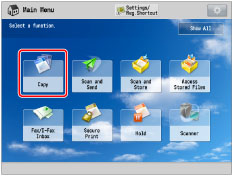2.
Press [Copy Ratio] → [Poster].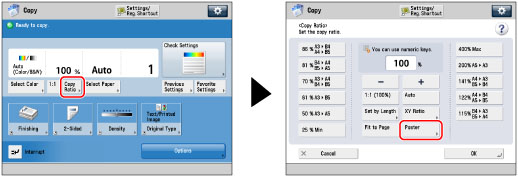3.
Press [Copy Ratio] → [Next].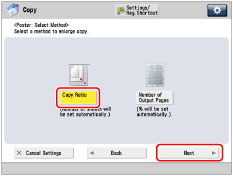4.
Use the numeric keys or press [-] or [+] on the touch panel display to enter a copy ratio → press [OK].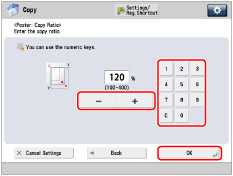5.
Press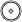NOTE If you press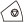while copying is in progress to cancel the copy job and then pressagain, copying resumes from the first sheet of the divided image.

## Selecting the Number of Output Pages

You can enlarge an original in the Poster mode by setting the number of output pages that the original image should be copied over.
1.
Press→ [Copy].2.
Press [Copy Ratio] → [Poster].3.
Press [Number of Output Pages] → [Next].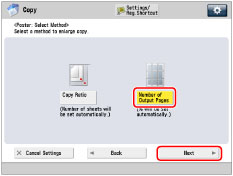4.
Press [Change].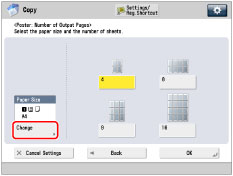If you are not changing the paper size, proceed to step 6.
5.
Select the desired paper size → press [OK].
If you select the Stack Bypass-C, the screens for specifying the paper size and paper type in the Stack Bypass-C are displayed.
6.
Select the number of output pages → press [OK].
You can select , , , or  for the number of pages.
Refer to the table under "Determining the Copy Ratio and Number of Sheets Needed" as a guideline for specifying the number of output pages.
 NOTE The number of output pages that you have selected is used as a guideline. The actual number of output pages maybe less than what you have selected, depending on a combination of factors that determine the most appropriate number of pages. These factors include the size of the original, the selected paper size, and the enlargement ratio.
7.
Press.
 NOTE If you presswhile copying is in progress to cancel the copy job and then pressagain, copying resumes from the first sheet of the divided image.
3U19-0WS